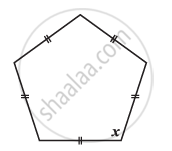# Find the Angle Measure X in the Given Figure - 4 - Mathematics

Find the angle measure x in the given Figure#### Solution

Sum of the measures of all interior angles of a pentagon is 540º.

5x = 540°

x = 108°

Concept: Angle Sum Property of a Quadrilateral
Is there an error in this question or solution?

#### APPEARS IN

NCERT Class 8 Maths